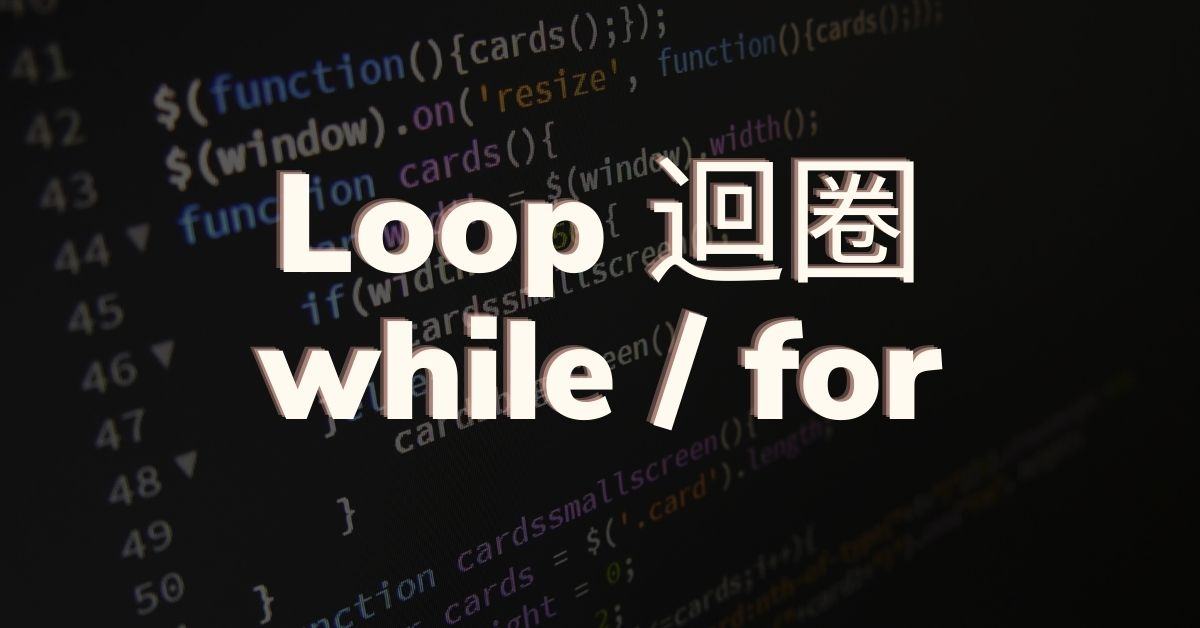# Javascript 迴圈（loop），學習 while / for的用法• while：只要條件有效，就持續執行
• for：控制執行次數

### 只要條件有效，就持續執行：While

```let x = 1
while (x <= 3) {
console.log(`I have looped \${x} time(s)...`)
x++
}
console.log('Finished!')```

• 關鍵字`while`
• 條件：在 `( )` 裡面定義條件，只要條件表達式 (expression) 回傳 true，就會執行 `{}` 裡的程序；
• 執行程序：將你希望重複的動作定義在 `{ }`之中。 使用 while 時需要設定一組條件式，這裡的使用方式和 if-else 的條件式相同，需要注意的地方也一樣。

#### do while 迴圈

```var times = 1;
do {
console.log(times);
times++;
} while (times < 5)
```

#### 小心無窮迴圈！

```while (true) {
console.log('This loop is running.....')
}```

3分鐘小測驗，找到自己學習全端開發的入口

## 控制執行次數：for 迴圈

``````for (let x = 1; x <= 3; x++ ) {
console.log(`I have looped \${x} time(s)...`)
}
console.log('Finished!')
``````

• 關鍵字`for`
• 條件：在 `( )` 裡面需要定義三種資訊：
1. 宣告索引變數，請注意需要關鍵字如 `let x = 1`
2. 停止條件，如 `x <= 3`，在此條件為 true 之前，迴圈都會執行；
3. 每次迭代的變化，如 `x++` 讓迴圈每執行一次，做為索引的變數 `x` 就會加 1。
• 執行程序：將你希望重複的動作定義在 `{ }`之中。

## For 迴圈與陣列

``````const weekDays = ['Mánudagur', 'Þriðjudagur', 'Miðvikudagur', 'Fimmtudagur', 'Föstudagur', 'Laugardagur', 'Sunnudagur' ]
``````

``````for (let x = 0; x < weekDays.length; x++) {
console.log(weekDays[x])
}
``````

``````for (let x = weekDays.length - 1; x >= 0 ; x--) {
console.log(`\${weekDays[x]} is day \${x + 1}`)
}
``````

## 跳脫迴圈：break

``````let items = ['lemons','bananas','stem ginger','<malicious code>','cocoa nibs']

let items = ['lemons','bananas','stem ginger','<malicious code>','cocoa nibs']
for (i = 0; i < items.length; i++) {
console.log(items[i])
}
``````

• 用 `"<"` 當成檢查的目標，如果 `"<"` 存在，就表示遇到惡意的內容，我們會使用`_string_.indexOf(_string_)` 方法，它會在一個字串中搜尋另一個字串，如果它沒找到相對應的字串，會回傳 -1；
• 跳出迴圈，使用 break

``````const items = ['lemons','bananas','stem ginger','<malicious code>','cocoa nibs']
let count = 0;
for (i = 0; i < items.length; i++) {
if (items[i].indexOf('<') >= 0) break
console.log(items[i])
count++
}
console.log(`There are \${items.length} items in the list. The app accepted \${count} of them. `)
``````